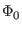Next: Vector Characteristics Up: Plots and Vectors Previous: Plots and Vectors   Contents   Index

The constants Plot

The following values are defined in a plot named constants''. This is the default plot if no rawfile has been loaded and no simulation has been run. These constants are visible no matter what the current plot is, but they are overridden by a vector with the same name in the current plot. The constants plot can not be deleted, and its vectors are read-only. The values are in MKS units.

 boltz Boltzmann's constant (1.38062e-23 joules/degree kelvin) const_c The speed of light (2.997925e8 meters/second) const_e The base of natural logarithms (2.71828182844590452353) echarge The charge on an electron (1.60219e-19 coulombs) false False value (0) const_j The square root of -1, can be expressed as (0,1) kelvin Absolute 0 in Centigrade (-273.15 degrees) no False value (0) phi0 The flux quantum (Planck's constant over twice echarge) phi0_2pi Value of the flux quantum normalized to 2pi(3.14159265358979323846) planck Planck's constant (6.62620e-34 joule-seconds) true Truth value (1) yes Truth value (1)Next: Vector Characteristics Up: Plots and Vectors Previous: Plots and Vectors   Contents   Index
Stephen R. Whiteley 2019-06-03